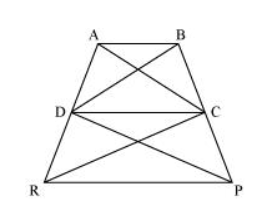# In the given figure, ar (DRC) = ar (DPC) and ar (BDP) = ar (ARC).

Question. In the given figure, $\operatorname{ar}(\mathrm{DRC})=\operatorname{ar}(\mathrm{DPC})$ and ar $(\mathrm{BDP})=\operatorname{ar}(\mathrm{ARC}) .$ Show that both the quadrilaterals $A B C D$ and $D C P R$ are trapeziums.

Solution:It is given that

Area $(\Delta \mathrm{DRC})=$ Area $(\Delta \mathrm{DPC})$

As $\triangle \mathrm{DRC}$ and $\triangle \mathrm{DPC}$ lie on the same base $D C$ and have equal areas, therefore, they must lie between the same parallel lines.

$\therefore \mathrm{DC} \| \mathrm{RP}$

Therefore, DCPR is a trapezium.

It is also given that

Area $(\Delta \mathrm{BDP})=$ Area $(\Delta \mathrm{ARC})$

$\Rightarrow$ Area $(B D P)-$ Area $(\Delta D P C)=\operatorname{Area}(\Delta A R C)-\operatorname{Area}(\Delta D R C)$

$\Rightarrow$ Area $(\Delta B D C)=$ Area $(\triangle A D C)$

Since $\triangle B D C$ and $\triangle A D C$ are on the same base $C D$ and have equal areas, they must lie between the same parallel lines.

$\therefore A B \| C D$

Therefore, ABCD is a trapezium.